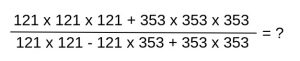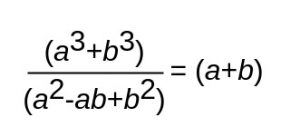IBM Placement Paper | Quantitative Analysis Set – 1

• Last Updated : 09 Nov, 2021

This is an IBM model paper for Quantitative Aptitude. This placement paper will cover aptitude that is asked in IBM placements and also strictly follows the pattern of questions asked in IBM papers. It is recommended to solve each one of the following questions to increase your chances of clearing the IBM placement.1. Find the greatest number that will divide 355, 54 and 103 so as to leave the same remainder in each case.

1. 4
2. 7
3. 9
4. 13

7

Explanation:

Required number = H.C.F. of |a -b|, |b – c| and |c – a|
= H.C.F. of |355 – 54|, |54 – 103| and |103 – 355|
= 301, 49, 252
= 7

2. Six bells commence tolling together and toll at intervals of 3, 6, 9, 12, 15 and 18 seconds respectively. In 60 minutes, how many times do they toll together?

1. 10
2. 20
3. 21
4. 25

21

Explanation:

L.C.M. of 3, 6, 9, 12, 15 and 18 is 180.
So, the bells will toll together after every 180 seconds(3 minutes).
In 60 minutes, they will toll together (60/3)+1 = 21 times.

3. The smallest 5 digit number exactly divisible by 11 is:

1. 11121
2. 11011
3. 10010
4. 11000

10010

Explanation:

The smallest 5-digit number 10000.

10000 when divided by 11, leaves a remainder of 1

Hence add (11 – 1) = 10 to 10000
Therefore, 10010 is the smallest 5 digit number exactly divisible by 114.

1. 474
2. 534
3. 500
4. 368

474

Explanation:

AsTherefore the given expression = (121 + 353) = 474

5. What decimal of 10 hours is a minute?

1. 0.025
2. 0.256
3. 0.0027
4. 0.00126

0.0027

Explanation:

Decimal of 10 hours in a minute
= 10 / (60 x 60)
= 0.0027

6. ‘A’ can do a work in 10 days and ‘B’ in 15 days. If they work on it together for 3 days, then the work that is left is :

1. 10%
2. 20%
3. 40%
4. 50%

50%

Explanation:

Let the total work to be done is, say, 30 units.

A does the work in 10 days,
So A’s 1-day work = (30 / 10) = 3 units

B does the work in 15 days,
So B’s 1-day work = (30 / 15) = 2 units

Therefore, A’s and B’s together 1-day work = (3 + 2) = 5 units

In 3 days,
work done = 5 * 3 = 15 units
amount of work left = 30 – 15 = 15 units

Therefore the % of work left after 3 days = (15 / 30) * 100% = 50%

7. A pump can fill a tank with water in 1 hour. Because of a leak, it took 1.5 hours to fill the tank. The leak can drain all the water of the tank in:

1. 2 hours
2. 2.5 hours
3. 3 hours
4. 3.5 hours

3 hours

Explanation:

Pump fills the tank in 1 hour

Time taken by Pump to fill due to leak = 1.5 hour
Therefore, in 1 hour, the amount of tank that the Pump can fill at this rate = 1 / (1.5) = 2/3

Amount of water drained by the leak in 1 hour = (1 – (2/3)) = 1/3

Therefore, the tank will be completely drained by the leak in (1 / (1/3)) = 3 hours

8. 2 pipes A and B can fill a tank in 20 minutes and 30 minutes respectively. Both pipes are opened. The tank will be filled in just 15 minutes, if the B is turned off after:

1. 5 min
2. 6.5 min
3. 7 min
4. 7.5 min

7.5 min

Explanation:

Let the total work to be done is, say, 60 units.

A fills the tank in 20 minutes,
So A’s 1-minute work = (60 / 20) = 3 units

B fills the tank in 30 minutes,
So B’s 1-minute work = (60 / 30) = 2 units

Therefore, A’s and B’s together 1-minute work = (3 + 2) = 5 units

Let the time when A and B both are opened be x minutes
and Since the total time taken to fill the tank is 15 minutes

Therefore, an expression can be formed as
5x + 3(15 – x) = 15
=> x = 7.5

Therefore, the B is turned off after 7.5 minutes

9. In an IPL match, the current run rate of CSK is 4.5 in 6 overs. What should be the required run rate of CSK inorder to achieve the target of 153 against KKR?

1. 7
2. 8
3. 8.5
4. 9

9

Explanation:

Current run rate = 4.5 in 6 overs

Target = 153
Runs still required = 153 – 27 = 126
Overs left = 14

Therefore required run rate = 126 / 14 = 9

10. The average of 10 numbers is 0. Of them, how many can be smaller than zero, at most?

1. 0
2. 1
3. 9
4. 10

9

Explanation:

Let the 9 numbers be smaller than zero and let their sum be ‘s’

Now, in order to get the average 0, the 10th number can be ‘-s’

Therefore, average = (s + (-s))/10 = 0/10 = 0

11. Which is not the prime number?.

1. 43
2. 57
3. 73
4. 101

57

Explanation:

A positive natural number is called prime number if nothing divides it except the number itself and 1.
57 is not a prime number as it is divisible by 3 and 19 also, apart from 1 and 57.

12. If the average of four consecutive odd numbers is 12, find the smallest of these numbers?

1. 5
2. 7
3. 9
4. 11

9

Explanation:

Let the numbers be x, x+2, x+4 and x+6
Then (x + x + 2 + x + 4 + x + 6)/4 = 12
∴ 4x + 12 = 48
∴ x = 9

13. Two numbers are in the ratio of 2:9. If their H. C. F. is 19, numbers are:

1. 6, 27
2. 8, 36
3. 38, 171
4. 20, 90

38, 171

Explanation:

Let the numbers be 2X and 9X
Then their H.C.F. is X, so X = 19
∴ Numbers are (2×19 and 9×19) i.e. 38 and 171

14. HCF of two numbers is 11 and their LCM is 385. If the numbers do not differ by more than 50, what is the sum of the two numbers?

1. 132
2. 35
3. 12
4. 36

132

Explanation:

Product of numbers = LCM x HCF
=> 4235 = 11 x 385

Let the numbers be of the form 11m and 11n,
such that ‘m’ and ‘n’ are co-primes.
=> 11m x 11n = 4235
=> m x n = 35
=> (m, n) can be either of (1, 35), (35, 1), (5, 7), (7, 5).
=> The numbers can be (11, 385), (385, 11), (55, 77), (77, 55).

But it is given that the numbers cannot differ by more than 50.
Hence, the numbers are 55 and 77.
Therefore, sum of the two numbers = 55 + 77 = 132

15. A person employed a group of 20 men for a construction job. These 20 men working 8 hours a day can complete the job in 28 days. The work started on time but after 18 days, it was observed that two-thirds of the work was still pending. To avoid penalty and complete the work on time, the employer had to employ more men and also increase the working hours to 9 hours a day. Find the additional number of men employed if the efficiency of all men is the same.

1. 40
2. 44
3. 64
4. 80

44

Explanation:

Let the total work be 3 units and additional men employed after 18 days be ‘x’.
=> Work done in first 18 days by 20 men working 8 hours a day = (1/3) x 3 = 1 unit
=> Work done in last 10 days by (20 + x) men working 9 hours a day = (2/3) x 3 = 2 unit

Here, we need to apply the formula

M1 D1 H1 E1 / W1 = M2 D2 H2 E2 / W2,

where
M1 = 20 men
D1 = 18 days
H1 = 8 hours/day
W1 = 1 unit
E1 = E2 = Efficiency of each man
M2 = (20 + x) men
D2 = 10 days
H2 = 9 hours/day
W2 = 2 unit

So, we have
20 x 18 x 8 / 1 = (20 + x) x 10 x 9 / 2
=> x + 20 = 64
=> x = 44

Therefore, number of additional men employed = 44

16. Two outlet pipes A and B are connected to a full tank. Pipe A alone can empty the tank in 10 minutes and pipe B alone can empty the tank in 30 minutes. If both are opened together, how much time will it take to empty the tank completely?

1. 7 minutes
2. 7 minutes 30 seconds
3. 6 minutes
4. 6 minutes 30 seconds

7 minutes 30 seconds

Explanation:

Let the capacity of the tank be LCM(10, 30) = 30 units.
=> Efficiency of pipe A = 30 / 10 = 3 units / minute
=> Efficiency of pipe A = 30 / 30 = 1 units / minute
=> Combined efficiency of pipe A and pipe B = 4 units / minute

Therefore, time required to empty the tank
if both pipes work = 30 / 4 = 7 minutes 30 seconds

17. Three pipes A, B, and C are connected to a tank. Working alone, they require 10 hours, 20 hours and 30 hours respectively. After some time, A is closed and after another 2 hours, B is also closed. C works for another 14 hours so that the tank gets filled completely. Find the time (in hours) after which pipe A was closed.

1. 1
2. 1.5
3. 2
4. 3

2

Explanation:

Let the capacity of the tank be LCM (10, 20, 30) = 60
=> Efficiency of pipe A = 60 / 10 = 6 units / hour
=> Efficiency of pipe B = 60 / 20 = 3 units / hour
=> Efficiency of pipe C = 60 / 30 = 2 units / hour

Now, all three work for some time, say ‘t’ hours.
So, B and C work for 2 more hours after ‘t’ hours
and then, C works for another 14 hours.

=> Combined efficiency of pipe A, pipe B and pipe C = 11 units / hour
=> Combined efficiency of pipe B and pipe C = 5 units / hour

So, we have 11 x t + 5 x 2 + 14 x 2 = 60
=> 11 t + 10 + 28 = 60
=> 11 t = 60 – 38
=> 11 t = 22
=> t = 2

Therefore, A was closed after 2 hours.

18. Two cars with speed of 15 kmph and 30 kmph respectively are 100 km apart and face each other. The distance between them 5 minutes before crossing is

1. 2.75
2. 3.75
3. 4.75
4. 5.75

3.75

Explanation:

As the two cars are moving towards each other,
their relative speed will be = 15+30 = 45 kmph.

The distance between them 5 minutes before crossing
will be equal to the distance travelled by their relative speed in 5 minutes,
i.e. Required distance = Relative speed (in km per min) * time (in minutes) = (45/60)*5 = 3.75 km.

My Personal Notes arrow_drop_up
Recommended Articles
Page :